CS 5371 Theory of Computation (Fall 2007)

General Information

 Lecturer: 韓永楷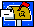wkhon @ cs.nthu.edu.tw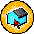EECS 741

 Tutor:   Shao-Chia Lu 呂紹甲g9562643@ oz.nthu.edu.twCSBB Lab

 Meeting Times: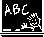Tue 1410—1500, Fri 1520—1710

 Announcement Jan 09: Solution of HW 5 [pdf] posted Jan 04: Revision Notes posted [pdf] Jan 04: Notes 24 posted for your interest [pdf] Dec 24: Solution of HW 4 [pdf] posted Useful Web Links v      Automata Theory by Ullman [link] v      Complexity Theory by Arora and Barak  [link] v      Computational Complexity by Goldreich  [link]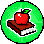Course Materials

 Lecture Topics Files 0 Overview [pdf] 1 Mathematics Review I  (Basic Terminology) [pdf] 2 Mathematics Review II (Common Proof Techniques) [pdf] 3 Automata Theory I (DFA and NFA) [pdf] 4 Automata Theory II (DFA = NFA, Regular Language) [pdf] 5 Automata Theory III (Non-regular Language, Pumping Lemma) [pdf] 6 Automata Theory IV (Reg Expression = NFA = DFA) [pdf] 7 Automata Theory V (CFG, CFL, CNF) [pdf] 8 Automata Theory VI (PDA, CFG = PDA) [pdf] 9 Automata Theory VII (Pumping Lemma, Non-CFL) [pdf] 10 Computability Theory I (Turing Machine) [pdf] 11 Computability Theory II (TM Variants, Church-Turing Thesis) [pdf] 12 Computability Theory III (Decidable Languages) [pdf] 13 Computability Theory IV (Undecidable Languages) [pdf] 14 Computability Theory V  (Prove by Reduction) [pdf] 15 Computability Theory VI (Post’s Correspondence, Reducibility) [pdf] 16 Complexity Theory I (Time Complexity) [pdf] 17 Complexity Theory II (Relationship Among Models) [pdf] 18 Complexity Theory III (P and NP) [pdf] 19 Complexity Theory IV (More on NP, NP-Complete) [pdf] 20 Complexity Theory V (Polynomial-Time Reducibility) [pdf] 21 Complexity Theory VI (More NP-Complete Problems) [pdf] 22 Complexity Theory VII (More NP-Complete Problems) [pdf] 23 Complexity Theory VIII (Space Complexity) [pdf] 24 Complexity Theory IX (PSPACE, L, NL) [pdf] *** The Revision [pdf]

 Tutorial Topics Related Files 1 Regular Languages, HW 1 Hints [pdf] 2 Pumping Lemma, HW 2 Hints [pdf] 3 HW 3 Hints [pdf]

 Assignment Topics Related Files 1 Regular Languages [pdf] [solution] 2 Context Free Grammar [pdf] [solution] 3 Turing Machines, Decidability [pdf] [solution] 4 Reducibility [pdf] [solution] 5 P, NP, and NP-complete [pdf] [solution]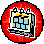Teaching Plan

 1 Regular Language DFA, NFA, Regular Expression, Pumping Lemma 2 Context-free Grammar CFG, PDA, Pumping Lemma 3 Turing Machines 4 Computability Halting Problem, Reduction Technique 5 Time Complexity Class P, NP, NP-Complete 6 Space Complexity Relationship between Time and Space 7 Intractability Problems Requiring More Time or Space 8 Advanced Topics Interactive Proof Systems, Cryptography, …

Last updated: 24 December 2007